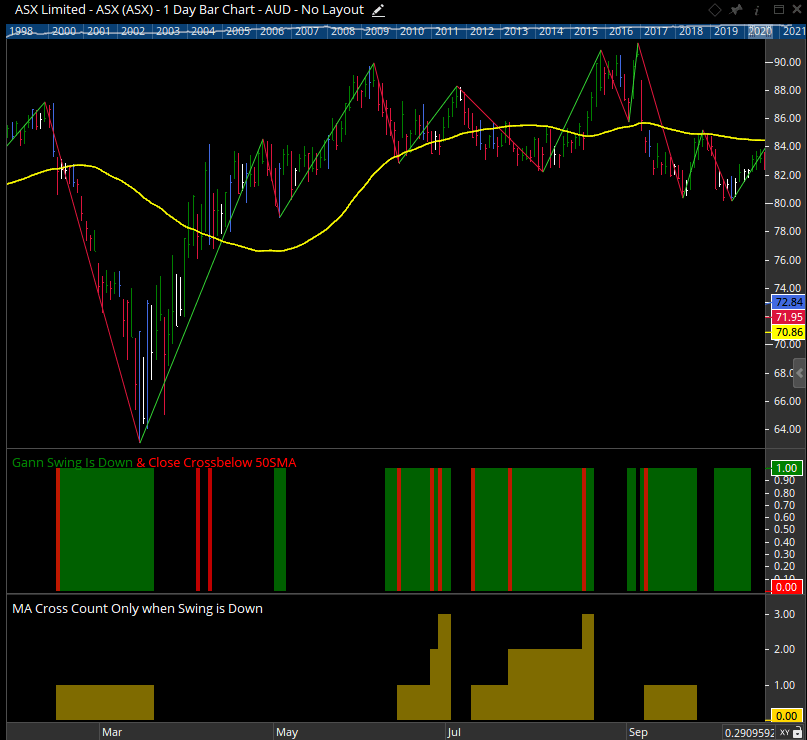# Accumulation Since Signal Function - ACCSINCESIGNAL()

Author: Optuma Team Last updated: Feb 8, 2023 18:11

## Overview

### ACCSINCESIGNAL(Source, Signal)

This function takes two scripts, the first script is the value which is accumulated and the second script is a Boolean value that signals a reset of the accumulation.

This example counts the number of positive days since the first trading day of 2019 using the BARDATE() function:

``````//Is it a positive day?
V1 = CHANGE()>0;

//Count how many times V1 was true since Jan 2nd 2019;
ACCSINCESIGNAL(V1, BARDATE()==43467)
``````

To calculate the % of positive days in 2019:

``````//Is it a positive day?
V1 = CHANGE()>0;

//All days will have a price > 0, so this will count the trading days;
V2 = CLOSE()>0;

//Count how many times V1 was true since Jan 2nd 2019;
V3=ACCSINCESIGNAL(V1, BARDATE()==43467);

//Count how many trading days since Jan 2nd 2019;
V4=ACCSINCESIGNAL(V2, BARDATE()==43467);

//Calculate the %
V3 / V4
``````

MFG has had 95 positive days at time of publishing, or 66.4% of the total number of trading days:Another example:

``````R1 = RSI() > 75;
R2 = RSI() < 72;
c1 = SWITCH(R1, R2);
c1_on = c1 and (OFFSET(c1, OFFSET=1) <= 0);
ACCSINCESIGNAL(RSI() , c1_on)
``````

This example script would accumulate the RSI values when the c1_on signal changes to a value of 1.Another Example:

This script counts the number of times the close crosses below a 50SMA while a Gann Swing is turned down. The count resets each time a the swing turns down, but is setup so that any cross that occurs during an upswing is not counted.

``````//Set Base Swing
V1 = GANNSWING(SWINGCOUNT=3);

//Set MA
V2 = MA(BARS=50) ;

//Find where the Swing Turns Down
V3 = V1 TurnsDown ;

//Find where the Close Crosses Below the 50SMA when the swing is down
V4 = CLOSE() CrossesBelow V2 and V1 IsDown;

//Count the signals
V5 = ACCSINCESIGNAL(V4,V3);

//Only include signals found while the swing is down
IF(V1 IsDown,V5,0)
``````

Here is how the above script would show on a chart (bottom Show View)…Red shaded zone shows where the close crosses below the 50SMA. The green shaded zone shows the the down swing area (from the point the down swing is confirmed). The script only counts red zones that occur within a green zone.